# Potential Energy Stored in a Spring

Definition: The spring constant, k , is a measure of the stiffness of a spring (large k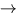stiff spring, small ksoft spring).

To compress a spring by a distance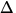x we must apply a force F ext = kx . By Newton's 3rd law, if we hold a spring in a compressed position, the spring exerts a force Fs = - kx . This is called a linear restoring force because the force is always in the opposite direction from the displacement.

Note:

• The sign of Fs shows that the spring resists attempts to compress or stretch it; therefore Fs is a restoring force.

For Example: In Figure (5.2a)x = xf - xi = - 5 which gives Fs = - k(- 5) = 5k . This force is positive and therefore directed to the right. This means that the spring resists the compression. In Figure (5.2b)x = xf - xi = 3 which gives Fs = - 3k . The negative sign indicates that the force is to the left and that the spring resists the stretching.• The farther we compress or stretch the spring, the greater the restoring force.
• We usually define xi = 0 and xf = x which gives Fs = - kx . This is called Hooke's law.

To find the potential energy stored in a compressed (or stretched) spring, we calculate the work to compress (or stretch) the spring: the force to compress a spring varies from F ext = F0 = 0 (at xi = 0 ), to F ext = Fx = kx (at xf = x ). Since force increases linearly with x , the average force that must be applied is=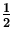(F0 + Fx) =kx
The work done by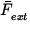is W =x =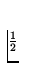kx 2. This work is stored in the spring as potential energy:
 PEs =kx 2. (4)
Note:
• PEs = 0 when x = 0 (at equilibrium).
• PEs always > 0 when the spring is not in equilibrium.
• PEs is the same if x =xf (same PEs for equal expansion or compression).Next: Choosing a Coordinate System Up: Work and Energy Previous: Gravitational Potential Energy